SCERT AP 7th Class Maths Solutions Pdf Chapter 4 Lines and Angles Unit Exercise Questions and Answers.

## AP State Syllabus 7th Class Maths Solutions 4th Lesson Lines and Angles Unit Exercise

Question 1.
Find the complementary, supplementary and conjugate angle of 36°.
Complementary angle of 36° is 90° – 36° – 54°
Supplementary angle of 36° is 180° – 36° = 144°
Conjugate angle of 36° is 360° – 36° = 324°

Question 2.
Observe the figure and write any 4 pairs of adjacent angles.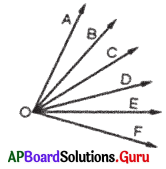Adjacent angle of ∠AOB is ∠BOC.
Adjacent angle of ∠BOC is ∠COD.
Adjacent angle of ∠COD is ∠DOE.
Adjacent angle of ∠DOE is ∠EOF.

Question 3.
In the given figure the lines l and m intersect at O. Find x.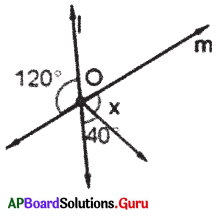Given l and m intersecting at O.
x° + 40° = 120° (vertically opposite angles)
x + 40° – 40°= 120° – 40°
∴ x = 80°Question 4.
In the given figure $$\overline{\mathbf{A E}}$$ is a straight line. If the ratio of angles ∠1, ∠2, ∠3, ∠4 in the given figure is 1:2 :3 : 4, then find the angles.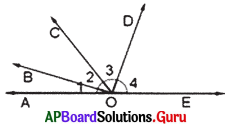Given $$\overline{\mathbf{A E}}$$ is a straight line.
Ratio of angles ∠1, ∠2, ∠3, ∠4 is 1 : 2 : 3 : 4 that is 1x: 2x: 3x: 4x

The sum of the angles at a point on the same side of the line is 180°
∠1 + ∠2 + ∠3 + ∠4 = 180°
⇒ 1x + 2x + 3x + 4x = 180°
⇒ 10x = 180
⇒ $$\frac{10 x}{10}=\frac{180}{10}$$
∴ x = 18°
2x = 2 × 18° = 36°
3x = 3 × 18° = 54°
4x = 4 × 18° = 72°
Therefore the angles are 18°, 36°, 54°, 72°.

Question 5.
Write any two examples for linear pair of angles in your surroundings.
Electric pole, Tree/Pen stand, etc.

Question 6.
Mani said, “Two obtuse angles can form a pair of conjugate angles.” Do you agree? Justify your answer.
Obtuse angle is always less than 180°. Sum of two obtuse angles is less than 360°.
So, I do not agree, that two obtuse angles cannot form a pair of conjugate angles.

Question 7.
Draw a pair of adjacent angles which are not supplementary to each other.
(i)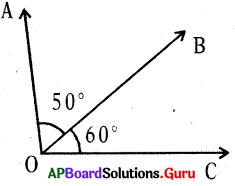∠AOB and ∠BOC are adjacent angles.
∠AOB + ∠BOC = 50° + 60°
= 110° ≠ 180°

∠AOB and ∠BOC are not supplementary.
(ii)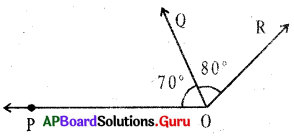∠POQ and ∠QOR are adjacent angles.
∠POQ + ∠QOR = 70° + 80°
= 150° ≠ 180°
∠POQ and ∠QOR are not supplementary.

Question 8.
In the figure, if l ∥ m, t is a transversal. Find ∠1 and ∠2.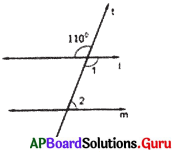Given l ∥ m and t is a transversal.
∠1 = 110° (vertically opposite angles)
∠1 + ∠2 = 180° (co-interior angles are supplementary)
110° + ∠2 = 180°
110° + ∠2 – 110° = 180° – 110°
∠2 – 70°
∠1 = 110° and ∠2 = 70°Question 9.
A line p intersects two lines l and m at two distinct points. Observe the figure and fill in the blanks :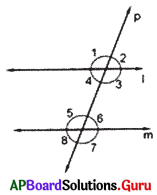(i) The line ‘p’ is known as ________, ________
(ii) ∠1 and ∠5 is a pair of ________ angles.
(iii) ∠4 and ∠6 is a pair of ________ angles.
(iv) ∠3 and ∠6 is a pair of ________ angles.
(i) transversal line,
(ii) corresponding
(iii) alternate interior
(iv) co-interior

Question 10.
In the given figure $$\overrightarrow{\mathbf{C F}} \| \overrightarrow{\mathbf{B D}}, \overrightarrow{\mathbf{B E}}$$ is transversal. ∠CAE = 135°, then find ∠ABD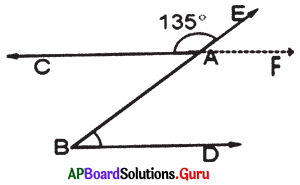Given $$\overrightarrow{\mathrm{CF}} \| \overrightarrow{\mathrm{BD}}$$ and $$\overrightarrow{\mathrm{BE}}$$ is transversal, ∠CAE — 135°